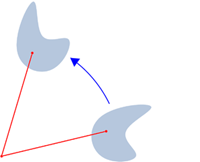# Rotation Worksheets

What is Geometric Rotation? We are familiar with four types of geometric transformations: Dilation: altering the size of the shape without altering its shape. Translation: sliding or moving the object without altering its size or rotating it. Reflection: flipping the object about the line of reflection. Rotation: rotating or spinning the shape about the point of rotation. Rotation is the movement of a shape or the object about a certain point of rotation. Rotation of the object is stated in degrees. If we get negative degrees, then the rotation is clockwise, and if the degrees are positive, then rotation is counterclockwise. In rotation, the shape or the object will not change the size, but its direction will be changed. The figure before rotation is referred to as the pre-image, and the rotated figure is referred to as an image.In the given figure, the pre-imaged is rotated to achieve the image. The size remains unchanged while the direction of the figure is altered. To show the rotation, we use the notation R (center, rotation) where rotation is the degrees at which the shape shifts, and the center is the point at which the shape rotates. We also describe rotation in terms of coordinate notation. That means that their coordinates on the plane are given.

• ### Basic Lesson

Guides students through identifying the center of rotation. An object and its rotation are the same shape and size, but the figures may be turned in different directions.

• ### Intermediate Lesson

Demonstrates how to find the angle of rotation.

• ### Independent Practice 1

A really great activity for allowing students to reinforce the concept of Rotation.

• ### Independent Practice 2

Students draw and Rotation in assorted problems. The answers can be found below.

• ### Homework Worksheet

Students are provided with problems to achieve the concepts of Rotation.

• ### Skill Quiz

This tests the students ability to master Rotation